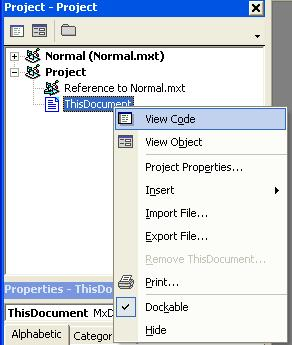HOW TO

# Use ArcObjects to get the x and y coordinates of points, polygon centroids, or line midpoints

## Summary

Instructions provided describe the steps using ArcObjects to get the x and y coordinates of points, polygon centroids, or line midpoints.

The code below generates a generic error if the data contains invalid geometry. If an error occurs while running the code, run the shapefile or personal geodatabase data through ArcToolbox's Repair Geometry command, located in the Data Management > Features toolset.

Point: If a point layer is selected, the fields added are called X_COORD and Y_COORD and are populated with the feature's x and y coordinates.

Line: If a line layer is selected, the fields added are called MID_X and MID_Y and contain the coordinates of the midpoint of each line.

Polygon: If a polygon layer is selected, the fields added are called CENTROID_X and CENTROID_Y and contain the coordinates of the centroid of each polygon.

## Procedure

The ArcObjects code below adds two new fields to a feature layer and can be applied with a point, line, or polygon layer selection.

1. Start ArcMap.
2. Add the feature class for which you want to calculate the coordinates. You must have write-access to the feature class.
3. Open the Visual Basic Editor.

In ArcMap, select Tools > Macros > Visual Basic Editor.

4. In the Project Explorer window, expand Project.mxt and select ArcMap Objects > ThisDocument then right-click and select View Code.`Note:Code in the Project's ThisDocument code module will only run in the current map document.  If you want to store the code in all your map documents open the Normal.mxt ThisDocument code module instead.`

5. Copy the following code into the code module.
`Code:Dim X_Pos As IntegerDim Y_Pos As IntegerDim fClass As IFeatureClassPublic Sub addCoords() ' Adds coordinate information to shapefile, coverage, ' and geodatabase feature class tables Dim mxDoc As IMxDocument Dim selectedLayer As ILayer Dim fLayer As IFeatureLayer  Set mxDoc = ThisDocument Set selectedLayer = mxDoc.selectedLayer If selectedLayer Is Nothing Then  MsgBox "Select a layer in the Table of Contents.", _         vbCritical, "No layer selected"  Exit Sub End If If Not TypeOf selectedLayer Is IFeatureLayer Then  MsgBox "Select a feature layer in the Table of Contents.", _         vbCritical, "Selected layer is not a feature layer"  Exit Sub End If Set fLayer = selectedLayer Set fClass = fLayer.FeatureClass Select Case fClass.ShapeType  Case esriGeometryPoint   If AddFields("X_Coord", "Y_Coord") = True Then    AddXY_Points   End If  Case esriGeometryPolyline   If AddFields("Mid_X", "Mid_Y") = True Then    AddXY_Lines  ' based on fromPoint of line.   End If  Case esriGeometryPolygon   If AddFields("Centroid_X", "Centroid_Y") = True Then    AddXY_Polygons  ' based on centroid of polygon.   End If  Case Else   MsgBox "This tool only works with point, line and polygons.", _          vbCritical, "Unsupported geometry type"   Exit Sub End SelectEnd SubPrivate Function AddFields(fieldName1 As String, _       fieldName2 As String) As Boolean        ' Add required fields that will store the coordinate information. On Error GoTo couldntAddFields Dim newField As IFieldEdit  ' Delete required field if it exists. If fClass.FindField(fieldName1) <> -1 Then  fClass.DeleteField fClass.Fields.Field(fClass.FindField(fieldName1)) End If  ' Create the required field. Set newField = New Field With newField  .Name = fieldName1  .Type = esriFieldTypeDouble End With fClass.AddField newField  ' Delete required field if it exists. If fClass.FindField(fieldName2) <> -1 Then  fClass.DeleteField fClass.Fields.Field(fClass.FindField(fieldName2)) End If  ' Create the required field. Set newField = New Field With newField  .Name = fieldName2  .Type = esriFieldTypeDouble End With fClass.AddField newField X_Pos = fClass.FindField(fieldName1) Y_Pos = fClass.FindField(fieldName2) If X_Pos = -1 Or Y_Pos = -1 Then  GoTo couldntAddFields End If AddFields = True Exit FunctioncouldntAddFields: MsgBox Err.Description & Chr(13) & "AddXY was not completed.", _        vbCritical, "Could not add required fields" AddFields = FalseEnd FunctionPrivate Sub AddXY_Points() ' Populate X_Coord and Y_Coord fields for each point. Dim fCursor As IFeatureCursor Dim aFeature As IFeature Dim thePoint As IPoint  Set fCursor = fClass.Update(Nothing, False) Set aFeature = fCursor.NextFeature Do Until aFeature Is Nothing  Set thePoint = aFeature.Shape  aFeature.Value(X_Pos) = thePoint.X  aFeature.Value(Y_Pos) = thePoint.Y  fCursor.UpdateFeature aFeature  Set aFeature = fCursor.NextFeature Loop MsgBox "Finished", vbInformation, "Add Coordinates"End SubPrivate Sub AddXY_Lines() ' Populate Mid_X and Mid_Y fields with the mid-point XY for each line. Dim fCursor As IFeatureCursor Dim aFeature As IFeature Dim theCurve As ICurve Dim thePoint As IPoint  Set thePoint = New Point Set fCursor = fClass.Update(Nothing, False) Set aFeature = fCursor.NextFeature Do Until aFeature Is Nothing  Set theCurve = aFeature.Shape  theCurve.QueryPoint 0, 0.5, True, thePoint  aFeature.Value(X_Pos) = thePoint.X  aFeature.Value(Y_Pos) = thePoint.Y  fCursor.UpdateFeature aFeature  Set aFeature = fCursor.NextFeature Loop MsgBox "Finished", vbInformation, "Add Coordinates"End SubPrivate Sub AddXY_Polygons() ' Populate Centroid_X and Centroid_X fields ' with the centroid for each polygon. Dim fCursor As IFeatureCursor Dim aFeature As IFeature Dim thePolygon As IArea Dim thePoint As IPoint  Set fCursor = fClass.Update(Nothing, False) Set aFeature = fCursor.NextFeature Do Until aFeature Is Nothing  Set thePolygon = aFeature.Shape  Set thePoint = thePolygon.Centroid  aFeature.Value(X_Pos) = thePoint.X  aFeature.Value(Y_Pos) = thePoint.Y  fCursor.UpdateFeature aFeature  Set aFeature = fCursor.NextFeature Loop MsgBox "Finished", vbInformation, "Add Coordinates"End Sub`

6. Close the Visual Basic Editor.
7. Run the code by clicking Tools > Macros > Macros, then change the 'Macros in:' to "Normal (Normal.mxt)", select "ThisDocument.addCoords" in the 'Macro name:' box, and then click Run. A message box will tell you when the code is finished running.

Article ID:000004898

Software:
• ArcMap 8 x
• ArcMap 9 x

Get help from ArcGIS experts

Contact technical support